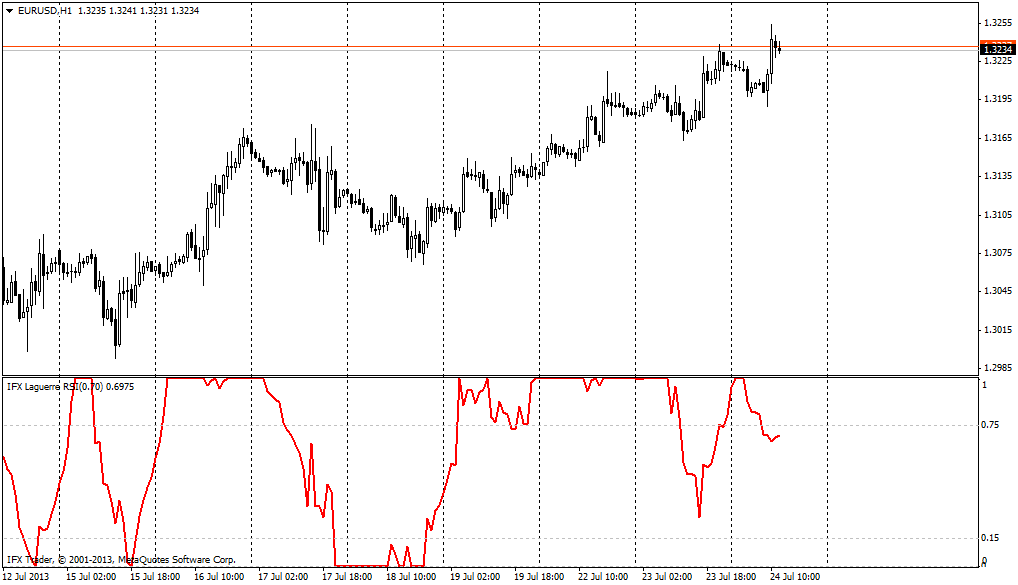## Instant account opening

Registration is not available for non-EU residents. Please, proceed to the website of the other member of the InstaForex group.#### Formula

L0:=((1-g)*C) + (g*PREV);

L1:=(-g*L0) + Ref(L0,-1) + (g*PREV);

L2:=(-g*L1) + Ref(L1,-1) + (g*PREV);

L3:=(-g*L2) + Ref(L2,-1) + (g*PREV);

cu:= If(L0 > L1, L0-L1,0) + If(L1 > L2, L1-L2,0) + If(L2 > L3, L2-L3,0);

cd:= If(L0 < L1, L1-L0,0) + If(L1 < L2, L2-L1,0) + If(L2 < L3, L3-L2,0).

As in case of many other oscillators, the main method of this indicator use is opening of buy orders after the level of indicator crosses above the level of 0.15 and opening of sell orders after the indicator crosses below the level of 0.75.

The major parameter of the RSI Laguerre indicator for MetaTrader is the factor gamma which equals 0.7 by default. It is important to note when the value which is less than this level (for instance, 0.5) is used, the indicator suggests rather aggressive trading with big number of trading signals, which nagatively influences the outcomes. High values of the indicator’s factor (more than 0.85) are unadvisable as overvaluation of the parameter may lead to delays in the work of the indicator.

The relevant distinguishing characteristic of the technical indicator RSI Laguerre is the situation when the indicator line takes minimal or maximal value moving strictly in horizontal way; it means powerful directed movement. Thus, if the indicator equals 1 and moves horizontally, strong trend movement, which is pointed up, prevails on the market. If the indicator equals zero and moves horizontally, then one should open sell deals in the developing downward movement.#### Parameters of InstaForex RSI Laguerre indicator

gamma = 0.7

Risk Warning: CFDs are complex instruments and come with a high risk of losing money rapidly due to leverage. 50% of retail investor accounts lose money when trading CFDs with this provider. You should consider whether you understand how CFDs work and whether you can afford to take the high risk of losing your money.
You are now leaving www.instaforex.eu, a website operated by INSTANT TRADING EU LTD